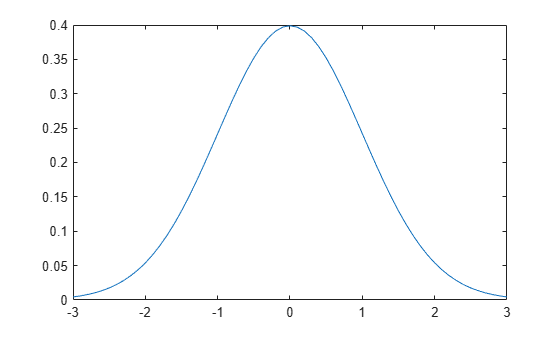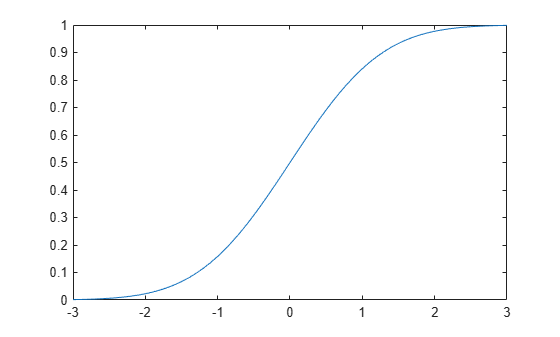Documentation

## Normal Distribution

### Overview

The normal distribution, sometimes called the Gaussian distribution, is a two-parameter family of curves. The usual justification for using the normal distribution for modeling is the Central Limit theorem, which states (roughly) that the sum of independent samples from any distribution with finite mean and variance converges to the normal distribution as the sample size goes to infinity.

Statistics and Machine Learning Toolbox™ offers several ways to work with the normal distribution.

### Parameters

The normal distribution uses these parameters.

ParameterDescriptionSupport
mu (μ)Mean$-\infty <\mu <\infty$
sigma (σ)Standard deviation$\sigma \ge 0$

The standard normal distribution has zero mean and unit standard deviation. If z is standard normal, then σz + µ is also normal with mean µ and standard deviation σ. Conversely, if x is normal with mean µ and standard deviation σ, then z = (xµ) / σ is standard normal.

#### Parameter Estimation

The maximum likelihood estimates (MLEs) are the parameter estimates that maximize the likelihood function. The maximum likelihood estimators of μ and σ2 for the normal distribution, respectively, are

$\overline{x}=\sum _{i=1}^{n}\frac{{x}_{i}}{n}$

and

${s}_{\text{MLE}}^{2}=\frac{1}{n}\sum _{i=1}^{n}{\left({x}_{i}-\overline{x}\right)}^{2}.$

$\overline{x}$ is the sample mean for samples x1, x2, …, xn. The sample mean is an unbiased estimator of the parameter μ. However, s2MLE is a biased estimator of the parameter σ2, meaning that its expected value does not equal the parameter.

The minimum variance unbiased estimator (MVUE) is commonly used to estimate the parameters of the normal distribution. The MVUE is the estimator that has the minimum variance of all unbiased estimators of a parameter. The MVUEs of the parameters μ and σ2 for the normal distribution are the sample mean and sample variance s2, respectively.

${s}^{2}=\frac{1}{n-1}\sum _{i=1}^{n}{\left({x}_{i}-\overline{x}\right)}^{2}$

To fit the normal distribution to data and find the parameter estimates, use normfit, fitdist, or mle.

• For uncensored data, normfit and fitdist find the unbiased estimates, and mle finds the maximum likelihood estimates.

• For censored data, normfit, fitdist, and mle find the maximum likelihood estimates.

Unlike normfit and mle, which return parameter estimates, fitdist returns the fitted probability distribution object NormalDistribution. The object properties mu and sigma store the parameter estimates.

For an example, see Fit Normal Distribution Object.

### Probability Density Function

The normal probability density function (pdf) is

$y=f\left(x|\mu ,\sigma \right)=\frac{1}{\sigma \sqrt{2\pi }}{e}^{\frac{-{\left(x-\mu \right)}^{2}}{2{\sigma }^{2}}},\text{ }\text{for}\text{\hspace{0.17em}}x\in ℝ.$

The likelihood function is the pdf viewed as a function of the parameters. The maximum likelihood estimates (MLEs) are the parameter estimates that maximize the likelihood function for fixed values of x.

For an example, see Compute and Plot the Normal Distribution pdf.

### Cumulative Distribution Function

The normal cumulative distribution function (cdf) is

$p=F\left(x|\mu ,\sigma \right)=\frac{1}{\sigma \sqrt{2\pi }}{\int }_{-\infty }^{x}{e}^{\frac{-{\left(t-\mu \right)}^{2}}{2{\sigma }^{2}}}dt,\text{ }\text{for}\text{\hspace{0.17em}}x\in ℝ.$

p is the probability that a single observation from a normal distribution with parameters μ and σ falls in the interval (-∞,x].

The standard normal cumulative distribution function Φ(x) is functionally related to the error function erf.

$\Phi \left(x\right)=\frac{1}{2}\left(1-\text{erf}\left(-\frac{x}{\sqrt{2}}\right)\right)$

where

$\text{erf}\left(x\right)=\frac{2}{\sqrt{\pi }}{\int }_{0}^{x}e{}^{-{t}^{2}}dt=2\Phi \left(\sqrt{2}x\right)-1.$

For an example, see Plot Standard Normal Distribution cdf

### Examples

#### Fit Normal Distribution Object

Load the sample data and create a vector containing the first column of student exam grade data.

load examgrades x = grades(:,1);

Create a normal distribution object by fitting it to the data.

pd = fitdist(x,'Normal')
pd = NormalDistribution Normal distribution mu = 75.0083 [73.4321, 76.5846] sigma = 8.7202 [7.7391, 9.98843] 

The intervals next to the parameter estimates are the 95% confidence intervals for the distribution parameters.

#### Compute and Plot the Normal Distribution pdf

Compute the pdf of a standard normal distribution, with parameters $\mu$ equal to 0 and $\sigma$ equal to 1.

x = [-3:.1:3]; y = normpdf(x,0,1);

Plot the pdf.

plot(x,y)#### Plot Standard Normal Distribution cdf

Create a standard normal distribution object.

pd = makedist('Normal')
pd = NormalDistribution Normal distribution mu = 0 sigma = 1 

Specify the x values and compute the cdf.

x = -3:.1:3; p = cdf(pd,x);

Plot the cdf of the standard normal distribution.

plot(x,p)#### Compare Gamma and Normal Distribution pdfs

The gamma distribution has the shape parameter $\mathit{a}$ and the scale parameter $\mathit{b}$. For a large $\mathit{a}$, the gamma distribution closely approximates the normal distribution with mean $\mu =\mathit{ab}$ and variance ${\sigma }^{2}=\mathit{a}{\mathit{b}}^{2}$. Compute the pdf of a gamma distribution with parameters A = 100 and B = 10. For comparison, also compute the pdf of a normal distribution with parameters mu = 1000 and sigma = 100.

x = gaminv((0.005:0.01:0.995),100,10); y_gam = gampdf(x,100,10); y_norm = normpdf(x,1000,100);

Plot the pdfs of the gamma distribution and the normal distribution on the same figure.

plot(x,y_gam,'-',x,y_norm,'-.') title('Gamma and Normal pdfs') legend('Gamma Distribution','Normal Distribution')#### Relationship Between Normal and Lognormal Distributions

If X follows the lognormal distribution with parameters µ and σ, then log(X) follows the normal distribution with mean µ and standard deviation σ. Use distribution objects to inspect the relationship between normal and lognormal distributions.

Create a lognormal distribution object by specifying the parameter values.

pd = makedist('Lognormal','mu',5,'sigma',2)
pd = LognormalDistribution Lognormal distribution mu = 5 sigma = 2 

Compute the mean of the lognormal distribution.

mean(pd)
ans = 1.0966e+03 

The mean of the lognormal distribution is not equal to the mu parameter. The mean of the logarithmic values is equal to mu. Confirm this relationship by generating random numbers.

Generate random numbers from the lognormal distribution and compute their log values.

rng('default'); % For reproducibility x = random(pd,10000,1); logx = log(x);

Compute the mean of the logarithmic values.

m = mean(logx)
m = 5.0033 

The mean of the log of x is close to the mu parameter of x, because x has a lognormal distribution.

Construct a histogram of logx with a normal distribution fit.

histfit(logx)The plot shows that the log values of x are normally distributed.

histfit uses fitdist to fit a distribution to data. Use fitdist to obtain parameters used in fitting.

pd_normal = fitdist(logx,'Normal')
pd_normal = NormalDistribution Normal distribution mu = 5.00332 [4.96445, 5.04219] sigma = 1.98296 [1.95585, 2.01083] 

The estimated normal distribution parameters are close to the lognormal distribution parameters 5 and 2.

#### Compare Student's t and Normal Distribution pdfs

The Student’s t distribution is a family of curves depending on a single parameter ν (the degrees of freedom). As the degrees of freedom ν goes to infinity, the t distribution approaches the standard normal distribution. Compute the pdfs for the Student's t distribution with the parameter nu = 5 and the Student's t distribution with the parameter nu = 25. Compute the pdf for a standard normal distribution.

x = -5:0.1:5; y1 = tpdf(x,5); y2 = tpdf(x,15); z = normpdf(x,0,1);

Plot the Student's t pdfs and the standard normal pdf on the same figure. The standard normal pdf has shorter tails than the Student's t pdfs.

plot(x,y1,'-.',x,y2,'--',x,z,'-') legend('Student''s t Distribution with \nu=5', ... 'Student''s t Distribution with \nu=25', ... 'Standard Normal Distribution','Location','best') title('Student''s t and Standard Normal pdfs')### Related Distributions

• Binomial Distribution — The binomial distribution models the total number of successes in n repeated trials with the probability of success p. As n increases, the binomial distribution can be approximated by a normal distribution with µ = np and σ2 = np(1–p).

• Birnbaum-Saunders Distribution — If x has a Birnbaum-Saunders distribution with parameters β and γ, then

$\frac{\left(\sqrt{x}{\beta }}-\sqrt{\beta }{x}}\right)}{\gamma }$

has a standard normal distribution.

• Chi-Square Distribution — The chi-square distribution is the distribution of the sum of squared, independent, standard normal random variables. If a set of n observations is normally distributed with variance σ2, and s2 is the sample variance, then (n–1)s2/σ2 has a chi-square distribution with n–1 degrees of freedom. The normfit function uses this relationship to calculate confidence intervals for the estimate of the normal parameter σ2 .

• Extreme Value Distribution — The extreme value distribution is appropriate for modeling the smallest or largest value from a distribution whose tails decay exponentially fast, such as, the normal distribution.

• Gamma Distribution — The gamma distribution has the shape parameter a and the scale parameter b. For a large a, the gamma distribution closely approximates the normal distribution with mean μ = ab and variance σ2 = ab2. The gamma distribution has density only for positive real numbers. See Compare Gamma and Normal Distribution pdfs.

• Half-Normal Distribution — The half-normal distribution is a special case of the folded normal and truncated normal distributions. If a random variable Z has a standard normal distribution, then $X=\mu +\sigma |Z|$ has a half-normal distribution with parameters μ and σ.

• Logistic Distribution — The logistic distribution is used for growth models and in logistic regression. It has longer tails and a higher kurtosis than the normal distribution.

• Lognormal Distribution — If X follows the lognormal distribution with parameters µ and σ, then log(X) follows the normal distribution with mean µ and standard deviation σ. See Relationship Between Normal and Lognormal Distributions.

• Multivariate Normal Distribution — The multivariate normal distribution is a generalization of the univariate normal to two or more variables. It is a distribution for random vectors of correlated variables, in which each element has a univariate normal distribution. In the simplest case, there is no correlation among variables, and elements of the vectors are independent, univariate normal random variables.

• Poisson Distribution — The Poisson distribution is a one-parameter discrete distribution that takes nonnegative integer values. The parameter, λ, is both the mean and the variance of the distribution. As λ increase, the Poisson distribution can be approximated by a normal distribution with µ = λ and σ2 = λ.

• Rayleigh Distribution — The Rayleigh distribution is a special case of the Weibull distribution with applications in communications theory. If the component velocities of a particle in the x and y directions are two independent normal random variables with zero means and equal variances, then the distance the particle travels per unit time follows the Rayleigh distribution.

• Stable Distribution — The normal distribution is a special case of the stable distribution. The stable distribution with the first shape parameter α = 2 corresponds to the normal distribution.

$N\left(\mu ,{\sigma }^{2}\right)=S\left(2,0,\frac{\sigma }{\sqrt{2}},\mu \right)\text{\hspace{0.17em}}.$

• Student's t Distribution — The Student’s t distribution is a family of curves depending on a single parameter ν (the degrees of freedom). As the degrees of freedom ν goes to infinity, the t distribution approaches the standard normal distribution. See Compare Student's t and Normal Distribution pdfs.

If x is a random sample of size n from a normal distribution with mean μ, then the statistic

$t=\frac{\overline{x}-\mu }{s/\sqrt{n}}$

where $\overline{x}$ is the sample mean and s is the sample standard deviation, has the Student's t distribution with n–1 degrees of freedom.

• t Location-Scale Distribution — The t location-scale distribution is useful for modeling data distributions with heavier tails (more prone to outliers) than the normal distribution. It approaches the normal distribution as the shape parameter ν approaches infinity.

 Abramowitz, M., and I. A. Stegun. Handbook of Mathematical Functions. New York: Dover, 1964.

 Evans, M., N. Hastings, and B. Peacock. Statistical Distributions. 2nd ed. Hoboken, NJ: John Wiley & Sons, Inc., 1993.

 Lawless, J. F. Statistical Models and Methods for Lifetime Data. Hoboken, NJ: Wiley-Interscience, 1982.

 Marsaglia, G., and W. W. Tsang. “A Fast, Easily Implemented Method for Sampling from Decreasing or Symmetric Unimodal Density Functions.” SIAM Journal on Scientific and Statistical Computing. Vol. 5, Number 2, 1984, pp. 349–359.

 Meeker, W. Q., and L. A. Escobar. Statistical Methods for Reliability Data. Hoboken, NJ: John Wiley & Sons, Inc., 1998.